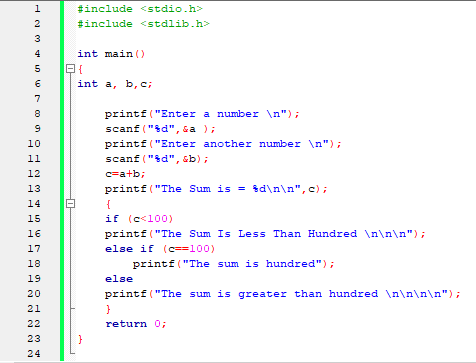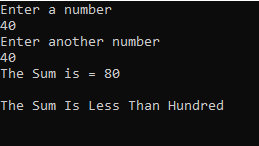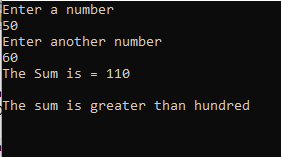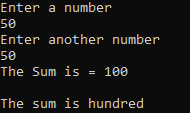# C Program to check sum is less than or greater than hundred

C Program to check sum is less than or greater than hundred
Input :-Output :-
1)2)3)Code :-

#include <stdio.h>
#include <stdlib.h>

int main()
{
int a, b,c;

printf("Enter a number \n");
scanf("%d",&a );
printf("Enter another number \n");
scanf("%d",&b);
c=a+b;
printf("The Sum is = %d\n\n",c);
{
if (c<100)
printf("The Sum Is Less Than Hundred \n\n\n");
else if (c==100)
printf("The sum is hundred");
else
printf("The sum is greater than hundred \n\n\n\n");
}
return 0;
}